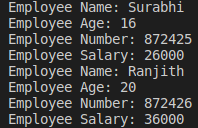# Polymorphism in Python

## Polymorphism in Python

In this article, I am going to discuss Polymorphism in Python i.e. Overloading and Overriding in Python with Examples. Please read our previous article where we discussed Super Function in Python. As part of this article, we are going to discuss the following pointers which are related to Polymorphism in Python.

1. What is Polymorphism in Python?
2. Types of Polymorphism in Python
3. Duck Typing Philosophy of Python
7. How we can handle overloaded method requirements in Python?
9. Overriding in Python
10. Method Overriding in Python
11. Constructor Overriding in Python
##### What is Polymorphism in Python?

The word ‘Poly’ means many and ‘Morphs’ means forms. The process of representing “one form in many forms” is called a polymorphism.

##### Types of Polymorphism in Python:

The following are the examples, or implementations, of polymorphism:

1.Duck Typing Philosophy of Python
3.Overriding
Method overriding
Constructor overriding

##### Duck Typing Philosophy of Python

Duck typing refers to the programming style, in which the object passed to a method supports all the attributes expected from it, at the runtime. The important thing here is not about the object type, but about what attributes and methods the object supports.

In duck typing, while creating a data, it’s not required to declare the argument type explicitly. At runtime, based on provided value the type will be considered automatically. Since Python is considered as a Dynamically Typed Programming Language, it follows Duck Typing.

“If it walks like a duck, swims like a duck, and quacks like a duck, then it probably is a duck.”

Duck Typing originates from the above saying. For example, let’s consider a class “Car” which has an attribute ‘engine_name’ and a method ‘start_engine’ which takes the ‘engine_name’ attribute and does the action of starting the engine. A ‘Truck’ is different from a ‘Car’ but for the duck typing it actually doesn’t matter. We can create any truck object for the ‘Car’ and use the ‘start_engine’ method to start it.

###### Program: Duck typing philosophy (demo1.py)
```class Duck:
def talk(self):
print("Quack.. Quack")
class Dog:
def talk(self):
print("Bow...Bow")
class Cat:
def talk(self):
print("Moew...Moew ")

def m(obj):
obj.talk()

duck = Duck()
m(duck)

cat = Cat()
m(cat)

dog = Dog()
m(dog)```

Output: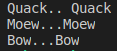In the above program, the function ‘m’ takes an object and calls for the talk() method of it. With duck typing, the function is not worried about what object type of object it is. The only thing that matters is whether the object has a method with name ‘talk()’ supported or not.

We can use the same operator or methods for different purposes. There are 3 types of overloading:

If we use the same operator for multiple purposes, then it is nothing but operator overloading.

1. ‘+’- addition operator can be used for Arithmetic addition and String concatenation as well
2. * multiplication operator can be used for multiplication for numbers and repetition for strings, lists, tuples etc

```print(10+20)
print("Python" + "Programming")
print([1,2,3]+[4,5,6])```

Output: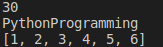```print(10*20)
print("Python"*3)
print([1,2,3]*3)```

Output: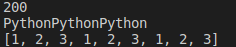Program: Addition operator in depth understanding (demo4.py)

```class Book:
def __init__(self, pages):
self.pages=pages

b1=Book(100)
b2=Book(200)
print(type(b1))
print(type(b2))

print(type(b1.pages))
print(type(b2.pages))

print(b1.pages + b2.pages)

Output: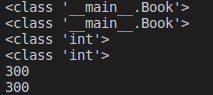We defined a class ‘Book’ and two objects ‘b1’ and ‘b2’ to it. We tried adding the pages attributes, which are integers, of the two objects in two different ways. One is the general way and the other is using ‘__add__’ method. Whenever we call for an operation using ‘+’ operator, this __add__ method is called by default. This is known as the magic method. There are many such magic methods for many operators.

###### Program: use + operator for user defined objects (demo5.py)
```class Book:
def __init__(self, pages):
self.pages=pages

b1=Book(100)
b2=Book(200)

print(b1 + b2)```

Output: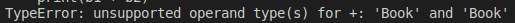In the above program, we are trying to add two user defined objects ‘b1’ and ‘b2’. The default, __add__ method, which will be called cannot perform the addition of two such objects. We can override the __add__ method in our class, in a way that it can act on user defined objects also. Overriding the methods will allow overloading of the + operator.

##### Magic Methods

For every operator Magic methods are available. To overload any operator, we should override that Method in our class. Internally + operator is implemented by using __add__() method. This method is called magic method for + operator.

```class Book:
def __init__(self, pages):
self.pages=pages
return self.pages + others.pages
b1=Book(100)
b2=Book(200)

print(b1 + b2)```

Output: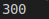List of operators and corresponding magic methods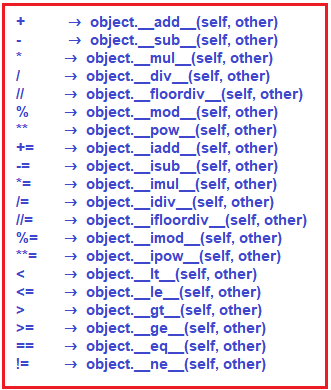Program: Using less than and greater than symbols on user defined objects (demo7.py)

```class Student:
def __init__(self, name, marks):
self.name=name
self.marks=marks

s1=Student("Samvida", 100)
s2=Student("Surya", 200)
print("s1>s2 =", s1>s2)
print("s1<s2 =", s1<s2)
print("s1<=s2 =", s1<=s2)
print("s1>=s2 =", s1>=s2)```

Output: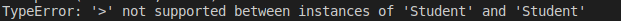```class Student:
def __init__(self, name, marks):
self.name=name
self.marks=marks
def __gt__(self, other):
return self.marks>other.marks
def __lt__(self, other):
return self.marks<=other.marks

s1=Student("Samvida", 100)
s2=Student("Surya", 200)
print("s1>s2 =", s1>s2)
print("s1<s2 =", s1<s2)```

Output: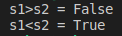If 2 methods have the same name but different types of arguments, then those methods are said to be overloaded methods.

But in Python Method overloading is not possible. If we are trying to declare multiple methods with the same name and different number of arguments, then Python will always consider only the last method.

Program: Methods names same and number of arguments are different (demo9.py)

```class Demo:
def m1(self):
print('no-arg method')
def m1(self, a):
print('one-arg method')
def m1(self, a, b):
print('two-arg method')

d= Demo()
d.m1()
#d.m1(10)
#d.m1(10,20)```

Output: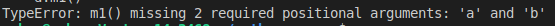```class Demo:
def m1(self):
print('no-arg method')
def m1(self, a):
print('one-arg method')
def m1(self, a, b):
print('two-arg method')

d= Demo()
#d.m1()
d.m1(10)
#d.m1(10,20)```

Output: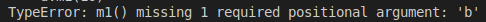```class Demo:
def m1(self):
print('no-arg method')
def m1(self, a):
print('one-arg method')
def m1(self, a, b):
print('two-arg method')

d= Demo()
#d.m1()
#d.m1(10)
d.m1(10,20)```

Output: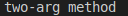###### Conclusion

If we are trying to declare multiple methods with the same name and different number of arguments, then Python will always consider only the method which was last declared. In the above program python will consider only the method with two arguments, the last method.

##### How we can handle overloaded method requirements in Python

Most of the time, if a method with a variable number of arguments is required then we can handle it with default arguments or with a variable length of argument methods.

###### Program: Default Arguments (demo11.py)
```class Demo:
def sum(self, a=None, b=None, c=None):
if a!=None and b!= None and c!= None:
print('The Sum of 3 Numbers:', a + b + c)
elif a!=None and b!= None:
print('The Sum of 2 Numbers:', a + b)
else:
print('Please provide 2 or 3 arguments')

d=Demo()
d.sum(10,20,30)
d.sum(10,20)
d.sum(10)```

Output: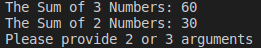Program: Variable length arguments (demo12.py)

```class Demo:
def sum(self, *a):
total=0
for x in a:
total=total+x
print('The Sum:', total)

d=Demo()
d.sum(10,20,30)
d.sum(10,20)
d.sum(10)```

Output: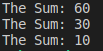Constructor overloading is also not possible in Python. If we define multiple constructors, only the last constructor will be considered.

```class Demo:
def __init__(self):
print('No-Arg Constructor')
def __init__(self, a):
print('One-Arg constructor')
def __init__(self, a, b):
print('Two-Arg constructor')

d1=Demo()
#d1=Demo(10)
#d1=Demo(10,20)```

Output: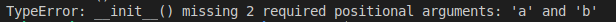```class Demo:
def __init__(self):
print('No-Arg Constructor')
def __init__(self, a):
print('One-Arg constructor')
def __init__(self, a, b):
print('Two-Arg constructor')

#d1=Demo()
d1=Demo(10)
#d1=Demo(10,20)```

Output: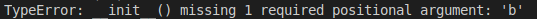```class Demo:
def __init__(self):
print('No-Arg Constructor')
def __init__(self, a):
print('One-Arg constructor')
def __init__(self, a, b):
print('Two-Arg constructor')

#d1=Demo()
#d1=Demo(10)
d1=Demo(10,20)```

Output: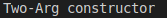In the above program only Two-Arg Constructor is available. But based on our requirement we can declare constructor with default arguments and variable length arguments.

###### Program: Constructor with Default Arguments (demo16.py)
```class Demo:
def __init__(self,a=None,b=None,c=None):
print(a)
print(b)
print(c)
d1=Demo(10, 20)```

Output: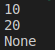Program: Constructor with Variable Length Arguments (demo17.py)

```class Demo:
def __init__(self, *a):
print('Constructor with variable number of arguments')

d1=Demo()
d2 = Demo(10)
d3 = Demo(10,20)
d4 = Demo(10,20,30)
d5 = Demo(10,20,30,40,50,60)```

Output: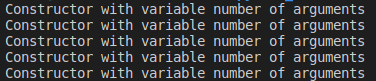##### Overriding in Python:

Overriding refers to the process of implementing something again, which already exists in parent class, in child class.

All the members available in the parent class, those are by-default available to the child class through inheritance. If the child class is not satisfied with parent class implementation, then child class is allowed to redefine that method in the child class based on its requirement. This concept is called overriding. Overriding concept applicable for both methods and constructors.

##### Method Overriding in Python:

Program: Method Overriding (demo18.py)

```class P:
def properties_status(self):
print('Money, Land, Gold')
def to_marry(self):
print('Anushka')
class C(P):
def study_status(self):
print("Studies done waiting for job")
def to_marry(self):
print('Megha')

c=C()
c.properties_status()
c.to_marry()
c.study_status()```

Output: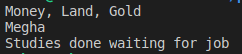##### Constructor Overriding in Python:

We have already discussed in detail about the constructor concepts in the inheritance chapter. Those concepts also include overriding concepts. Let’s remember some points from there:

1. If child class does not have constructor, then parent class constructor will be executed at the time of child class object creation.
2. If child class has a constructor, then child class constructor will be executed at the time of child class object creation.
3. From child class constructor we can call parent class constructor by using super() method
###### Program: Constructor Overriding (demo19.py)
```class Person:
def __init__(self, name, age):
self.name=name
self.age=age
class Employee(Person):
def __init__(self, name, age, eno, esal):
super().__init__(name, age)
self.eno=eno
self.esal=esal
def display(self):
print('Employee Name:', self.name)
print('Employee Age:', self.age)
print('Employee Number:', self.eno)
print('Employee Salary:', self.esal)

e1=Employee('Surabhi', 16, 872425,26000)
e1.display()
e2=Employee('Ranjith',20,872426,36000)
e2.display()```

Output: